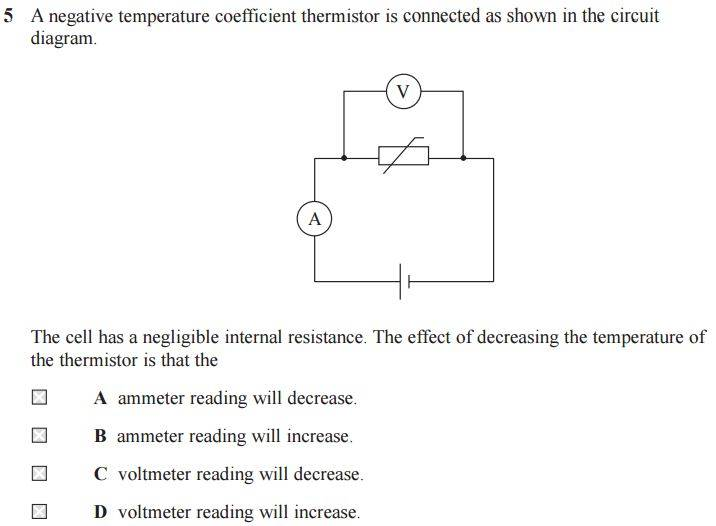# Current through voltmeter and ammeter

• ravsterphysics

#### ravsterphysics3. The Attempt at a Solution

As temp decreases, the resistance of the thermistor increases...doesn't this mean that the voltmeter will increase too since V=IR?

I know that the total resistance of the circuit has increased so less current, but I put down option D but the correct answer is A?

Suppose that the voltmeter and ammeter are ideal and the cell has emf V. If at some moment the thermistor has a resistance R, what voltage would the voltmeter measure across R?

•Albert Thomas
There's never any current through an ideal voltmeter, and never any voltage drop through an ideal ammeter.